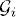# INDEPENDENCE

Independence is an important concept in probability theory. Intuitively, two events are independent if the occurrence of one event does not make the other happen more or less. In this chapter, we will introduce the concept of independence of events and random variables.

# 12.1 Basic Concepts and Facts

Definition 12.1 (Independent σ-Algebras). Let (Ω,, P) be a probability space. Sub-σ-algebrasofare considered independent if and only iffor all sets.

Let, i(an arbitrary index set) be an arbitrary family of sub-σ-algebras of. The are deemed independent if and only ...

Get Measure, Probability, and Mathematical Finance: A Problem-Oriented Approach now with the O’Reilly learning platform.

O’Reilly members experience live online training, plus books, videos, and digital content from nearly 200 publishers.